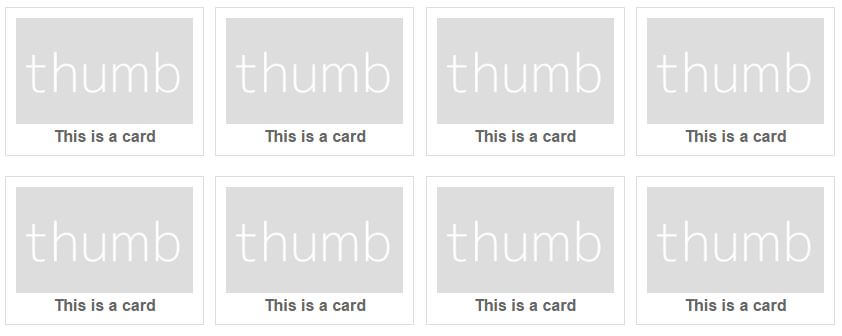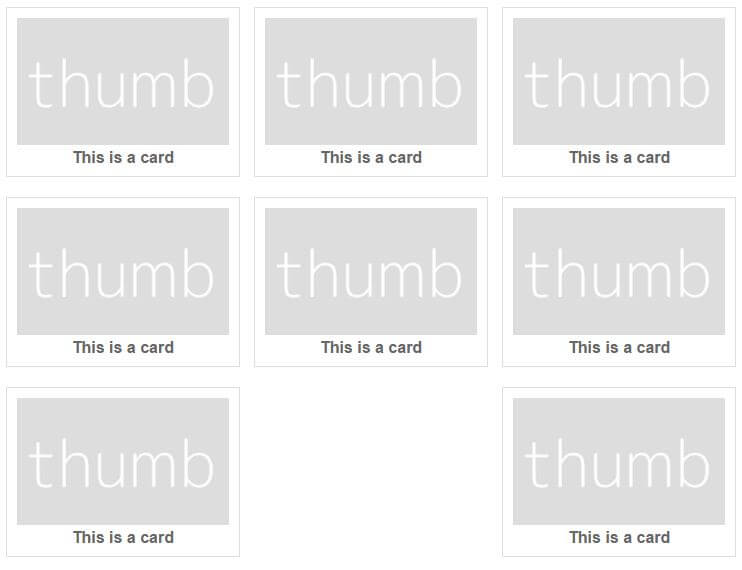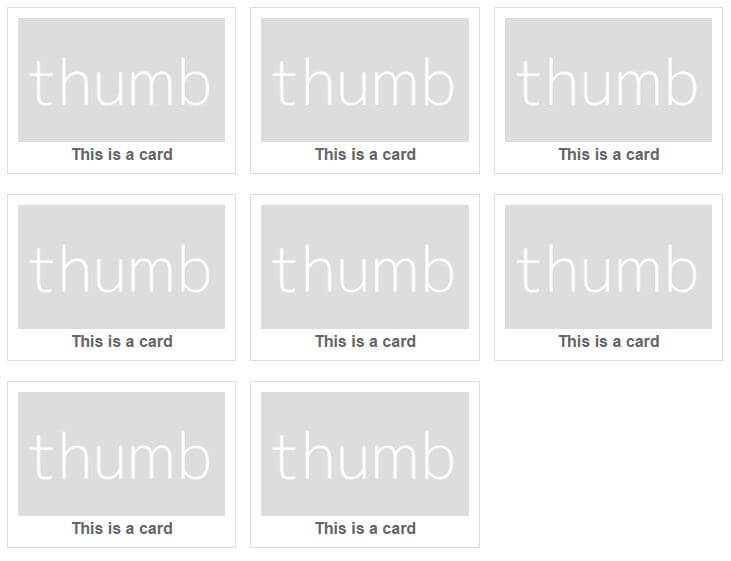# Flexbox space-between and the last row

I'm smitten with flexbox. To really get to grips with it I built a comprehensive, minimum styling site to really grasp how to do layout with it.

I created a 'card' layout with eight cards and had two rows of four. Looked great:So I tested the responsiveness and at my desired breakpoint the rows change to three cards (thanks to `flex-wrap`) and I was struck something that didn't look good:Despite a lot of playing around with flexbox properties and margins etc. I could not find a pure css solution so I had to embrace some 'progressive enhancement' and turn to JavaScript.

It struck me that in order to shift the last element along I would need an invisible element. Since I couldn't be certain of how many cards there would be, I'd need to add enough invisible elements to bring the total up to a number divisible by both three and four. The smallest number that divides by both is twelve.

The first function called takes an ID of the element that contains the cards. It then counts the child elements and passes that to the `checkCount()` function:

``````var container = document.getElementById(container_id);
var card_count = container.children.length;
checkCount(card_count, ...
``````

The `checkCount()` function takes the total number of cards and a callback function as arguments. By using the modulus operator (%) we can test to see if any remainder exists after dividing by twelve. Since a remainder other than zero is treated as true we know when we hit the right number when the if statement is false.

So if we do get a remainder, we increment the count and pass that to the same function. Hurrah for recursion!

``````function checkCount(current_total, next){
if(current_total % 12){ // 12 is smallest number that 3 and 4 go in to
current_total += 1; // Proper way to do ++
checkCount(current_total, next); // Recursion!
} else {
next(current_total);
}
}
``````

With the final count returned we can find the number of extra elements required by subtracting the original card count from it. Using this in a for loop we create elements with the class `dummy_card` and append them to the original container.

``````var dummy_element;
for(i=0; i<(final_count-card_count); i++){
dummy_element = document.createElement('div');
dummy_element.className = 'dummy_card';
container.appendChild(dummy_element);
}
``````

By giving the `dummy_card` a height of zero in the CSS it won't affect the look of the page. Although this approach does pollute the DOM a bit, by using empty div elements we don't affect the semantics. Seems to work.Here's the full code:

``````function checkCount(current_total, next){
if(current_total % 12){ // 12 is smallest number that 3 and 4 go in to
current_total += 1; // Proper way to do ++
checkCount(current_total, next); // Recursion!
} else {
next(current_total);
}
}